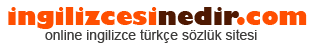ingilizce türkçe sözlük sitesine hoşgeldiniz.| Ana Sayfaİngilizce Türkçe Sözlükte Ara: Türkçe->İngilizce İngilizce->Türkçe * Aramak istediğiniz kelimeyi yazıp dil seçimini seçtikten sonra kelimenin anlamını öğrenebilirsiniz.

Kelime Anlamı

Türkçeden İngilizceye Çeviri Sonucu

bel Anlamı

• The Babylonian-Assyrian version of Baal, a common name for Marduk, chief god of Babylon , sometimes called Merodach by the Jews.
• A stylized creeper pattern.
• Named for Alexander Graham Bell, who did the original scientific investigations; Also see decibel.
• A title meaning Lord The Babylonian God Marduk was refered to as Bel.
• A measurement of sound intensity named in honor of Alexander Graham Bell First used to relate intensity to a level corresponding to hearing sensation.
• Equal to 10 decibels, see decibel.
• Belarus ).
• A dimensionless unit for expressing the ratio of two values of power, being the logarithm to the base 10 of the power ratio , is 10 times the logarithm to the base 10 of the power ratio A bel is 10 decibel ).
• A unit that represents the logarithm of the ratio of two levels The number of bels is equal to the logarithm sub 10 of P sub 1/P sub 2):2 logarithm sub 10 ; and 2 logarithm sub 10 See dB.
• A unit that represents the logarithm of the ratio of two levels The number of bels is equal to the logarithm 10 ; 2 logarithm 10 ; and 2 logarithm 10 See dB.
• The fundamental division of a logarithmic scale for expressing the ratio of two amounts of power, the number of bels denoting such a ratio being the logarithm to the base 10 of this ratio.
• A unit that represents the logarithm of the ratio of two levels.
• The base-10 logarithm of the ratio of two power values The basis for the more-common term decibel: One bel equals 10 decibels.
• Babylonian god of the earth; one of the supreme triad including Anu and Ea; earlier identified with En-lil.
• a logarithmic unit of sound intensity equal to 10 decibels.
• waist. loins. the small of the back. sperm. spade.
• Babylonian god of the earth; one of the supreme triad including Anu and Ea; earlier identified with En-lil a logarithmic unit of sound intensity equal to 10 decibels.
• The fruit is used medicinally, and the rind yields a perfume and a yellow dye.
• A thorny rutaceous tree of India, and its aromatic, orange-like fruit; called also Bengal quince, golden apple, wood apple.
• See Baal.
• The Babylonian name of the god known among the Hebrews as Baal.